# Maurer-Cartan form

(Redirected from Maurer–Cartan form)

A left-invariant-form on a Lie group, i.e. a differential formof degree 1 onsatisfying the condition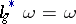for any left translation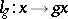,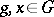. The Maurer–Cartan forms onare in one-to-one correspondence with the linear forms on the tangent space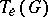at the point; specifically, the mapping which sends each Maurer–Cartan formto its valueis an isomorphism of the space of Maurer–Cartan forms onto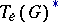. The differential of a Maurer–Cartan formis a left-invariant-form on, defined by the formula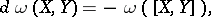(1)

whereare arbitrary left-invariant vector fields on. Suppose thatis a basis inand let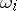,, be Maurer–Cartan forms such thatThen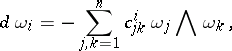(2)

where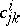are the structure constants of the Lie algebraofconsisting of the left-invariant vector fields on, with respect to the basisdetermined by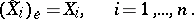The equalities (2) (or (1)) are called the Maurer–Cartan equations. They were first obtained (in a different, yet equivalent form) by L. Maurer . The formswere introduced by E. Cartan in 1904 (see ).

Letbe the canonical coordinates in a neighbourhood of the pointdetermined by the basis. Then the formsare written in the formin which the matrix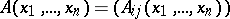is calculated by the formula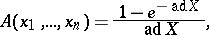whereand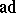is the adjoint representation of the Lie algebra.

Furthermore, letbe the-valued-form onwhich assigns to each tangent vector tothe unique left-invariant vector field containing this vector (is called the canonical left differential form). Then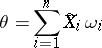and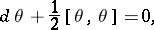which is yet another way of writing the Maurer–Cartan equations.